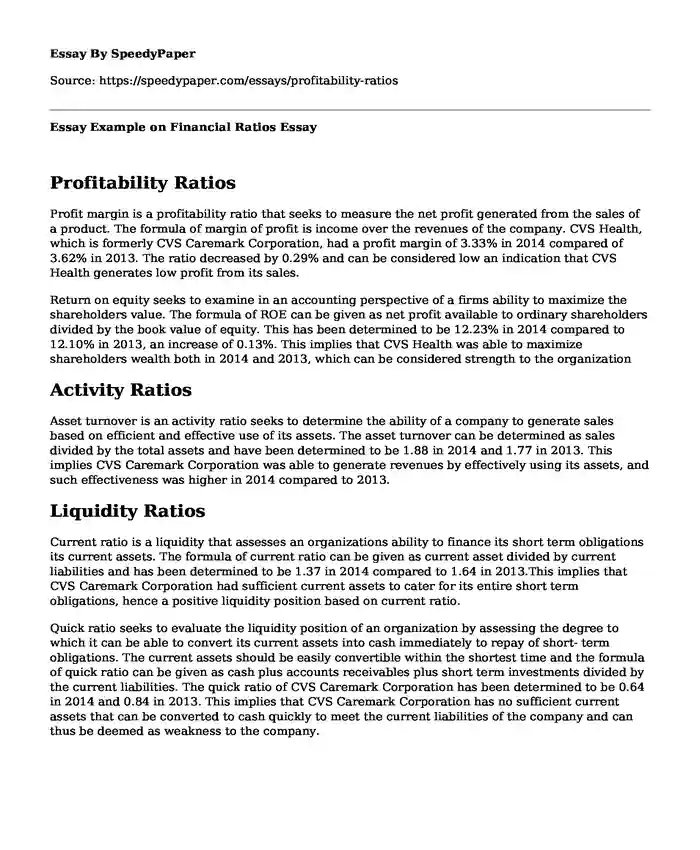# Essay Example on Financial Ratios

Published: 2020-06-10Categories: Business Financial management Pages: 3 Wordcount: 630 words
143 views

## Profitability Ratios

Profit margin is a profitability ratio that seeks to measure the net profit generated from the sales of a product. The formula of margin of profit is income over the revenues of the company. CVS Health, which is formerly CVS Caremark Corporation, had a profit margin of 3.33% in 2014 compared of 3.62% in 2013. The ratio decreased by 0.29% and can be considered low an indication that CVS Health generates low profit from its sales.

Is your time best spent reading someone else’s essay? Get a 100% original essay FROM A CERTIFIED WRITER!

Return on equity seeks to examine in an accounting perspective of a firms ability to maximize the shareholders value. The formula of ROE can be given as net profit available to ordinary shareholders divided by the book value of equity. This has been determined to be 12.23% in 2014 compared to 12.10% in 2013, an increase of 0.13%. This implies that CVS Health was able to maximize shareholders wealth both in 2014 and 2013, which can be considered strength to the organization

## Activity Ratios

Asset turnover is an activity ratio seeks to determine the ability of a company to generate sales based on efficient and effective use of its assets. The asset turnover can be determined as sales divided by the total assets and have been determined to be 1.88 in 2014 and 1.77 in 2013. This implies CVS Caremark Corporation was able to generate revenues by effectively using its assets, and such effectiveness was higher in 2014 compared to 2013.

## Liquidity Ratios

Current ratio is a liquidity that assesses an organizations ability to finance its short term obligations its current assets. The formula of current ratio can be given as current asset divided by current liabilities and has been determined to be 1.37 in 2014 compared to 1.64 in 2013.This implies that CVS Caremark Corporation had sufficient current assets to cater for its entire short term obligations, hence a positive liquidity position based on current ratio.

Quick ratio seeks to evaluate the liquidity position of an organization by assessing the degree to which it can be able to convert its current assets into cash immediately to repay of short- term obligations. The current assets should be easily convertible within the shortest time and the formula of quick ratio can be given as cash plus accounts receivables plus short term investments divided by the current liabilities. The quick ratio of CVS Caremark Corporation has been determined to be 0.64 in 2014 and 0.84 in 2013. This implies that CVS Caremark Corporation has no sufficient current assets that can be converted to cash quickly to meet the current liabilities of the company and can thus be deemed as weakness to the company.

## Solvency Ratios

Debt to- Asset ratio seeks to highlight the amount of debt financing available in the capital structure of a company. It shows the value the companys asset attributable to debt holders and equity holders. The formula of debt to -asset ratio can be given as total liabilities divided by the total assets and this has been determined to be 48.87% in 2014 and 49.96% in 2013. This implies that CVS Caremark Corporation is financed more by equity than by debt.

Debt to -Equity ratio seeks to analyze the degree to which assets of an organization have been financed by constituents of the capital structure, that is, shareholders equity and debt. It shows the amount of debt for every dollar of owners equity used to finance the organizations assets. The formula of debt- to equity ratio can be given as total liabilities divided by the total shareholders equity and this has been determined to be 0.96 in 2014 and 0.89 in 2013.This ratio implies that CVS Caremark Corporation finances most of its assets using equity and the increase in the ratio shows that in 2014, the company used more debt to finance assets/